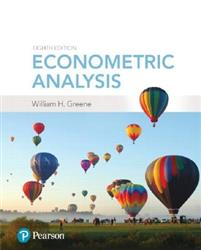## Examples Replications by Solomon Negash#### Part I The Linear Regression Model

CHAPTER 1 Econometrics [R]
CHAPTER 2 The Linear Regression Model [R]
CHAPTER 3 Least Squares Regression [R]
CHAPTER 4 Estimating the Regression Model by Least Squares
CHAPTER 5 Hypothesis Tests and Model Selection [R]
CHAPTER 6 Functional Form, Difference in Differences, and Structural Change
CHAPTER 7 Nonlinear, Semiparametric, and Nonparametric Regression Models
CHAPTER 8 Endogeneity and Instrumental Variable Estimation

#### Part II Generalized Regression Model and Equation Systems

CHAPTER 9 The Generalized Regression Model and Heteroscedasticity
CHAPTER 10 Systems of Regression Equations
CHAPTER 11 Models for Panel Data

#### Part III Estimation Methodology

CHAPTER 12 Estimation Frameworks in Econometrics
CHAPTER 13 Minimum Distance Estimation and the Generalized Method of Moments
CHAPTER 14 Maximum Likelihood Estimation
CHAPTER 15 Simulation-Based Estimation and Inference and Random Parameter Models
CHAPTER 16 Bayesian Estimation and Inference

#### Part IV Cross Sections, Panel Data, and Microeconometrics

CHAPTER 17 Binary Outcomes and Discrete Choice
CHAPTER 18 Multinomial Choices and Event Counts
CHAPTER 19 Limited Dependent Variables—Truncation, Censoring, and Sample Selection

#### Part V Time Series and Macroeconometrics

CHAPTER 20 Serial Correlation
CHAPTER 21 Nonstationary Data